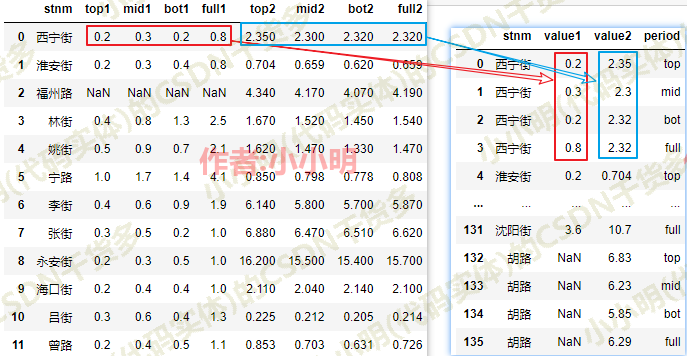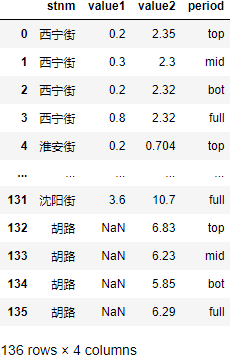``````import pandas as pd
import numpy as np

``````

``````result = []
for row in df.values:
result.append(
np.c_[
[row]*4,
row[1:5],
row[5:],
['top', 'mid', 'bot', 'full']
]
)
result = pd.DataFrame(np.vstack(result), columns=[
"stnm", "value1", "value2", "period"])
result
````````````def split_reshape_df(df, tags=['top', 'mid', 'bot', 'full']):
result = []
for row in df.values:
result.append(
np.c_[[row]*len(tags), row[1:len(tags)+1],
row[len(tags)+1:], tags]
)
result = pd.DataFrame(np.vstack(result), columns=[
df.columns, "value1", "value2", "period"])
return result
``````

`for row in df.values`取出了DataFream的Numpy对象并迭代出来每一行。

``````np.c_[row[1:5], row[5:]]
# 等价于：
np.column_stack([row[1:5], row[5:]])
# 等价于：
np.hstack([row[1:5, np.newaxis], row[5:, np.newaxis]])
# 等价于：
np.concatenate([row[1:5, np.newaxis], row[5:, np.newaxis]], axis=1)
``````

``````np.hstack([row[1:5, None], row[5:, None]])
``````

``````np.r_[result, result]
# 等价于：
np.row_stack([result, result])
# 等价于：
np.vstack([result, result])
# 等价于(concatenate的axis参数默认值为0，可以省略)：
np.concatenate([result, result])
``````